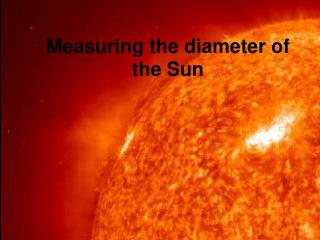DownloadDownload PresentationMeasuring the diameter of the Sun

# Measuring the diameter of the Sun

Download Presentation## Measuring the diameter of the Sun

- - - - - - - - - - - - - - - - - - - - - - - - - - - E N D - - - - - - - - - - - - - - - - - - - - - - - - - - -
##### Presentation Transcript

1. Measuring the diameter of the Sun

2. Aristarchus Aristarchus (310 B.C. - 230 B.C.) was a Greek astronomer and mathematician who was the first to propose a heliocentric model of the solar system placing the Sun and not the Earth at the centre of the universe. Aristarchus also calculated the size of the Moon and the size of and distance to the Sun.

3. Consider the previous diagram which represents the relative positions of the Sun, Moon and Earth during a first quarter moon. Aristarchus reasoned that during a first (or third) quarter moon, the angle between the Sun and Earth at the Moon is a right angle or900. • If you can then measure the angle between the Sun and the Moon, as shown in the previous diagram, it is possible to calculate the distance between the Earth and the Sun

4. What difficulties do you think Aristarchus encountered when working out his calculation ? • The measurement of the angle between the Sun and the Moon during first quarter phase was difficult when Aristarchus was alive due to the fact that the Sun is very far away and the angle between the Sun and the Moon is therefore very close to 900. In addition, it is very hard to determine when the Moon is in exactly quarter phase.

5. Activity: to measure the distance to the Sun: • Use a value of 89.8530 as the angle between the Sun and Moon. Call this angle . • Use 384,403 km for the distance from the Earth to the Moon • Calculate the distance to the Sun cosine() = (distance to moon)/(distance to Sun)

6. Activity – Measure the diameter of the Sun • In order to complete this activity we will construct a device called a “pinhole viewer” which is basically a pinhole camera without the photographic film.

7. Method 1) Find a suitable tube at least 600 mm in length 2) Place a piece of tin foil over one end and tape it in place over the edges 3) Using a pin or another sharp point, puncture the tin foil to produce a small hole. The maximum diameter of the hole depends on the tube length 4) Now tape some greaseproof paper on the other end of the tube. This will act as a screen.

8. For this method to work, light from the Sun must pass through the pinhole and fall on the greaseproof paper forming an image. • The measurements to be taken are 1) the diameter of the image of the Sun on the paper and 2) the distance from the pinhole to the paper, which is the length of the tube.

9. Calculate the diameter of the Sun using a value of 149,600,000 km for the Earth-Sun distance. Use the following formula: (Sun image diameter)/(Tube length) = (Actual Sun diameter)/(Actual Earth-Sun distance)

10. Using this method, we should get a value for the diameter of the Sun which is the right order of magnitude (~700,000 km).

11. This method can also be used to calculate the diameter of the Moon. • The Moon-Earth distance is 384,000 km. • Useful website: http://www.astro.washington.edu/labs/eratosthenes/index.html